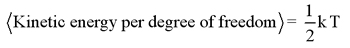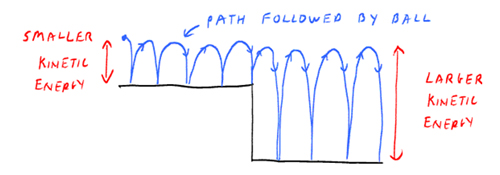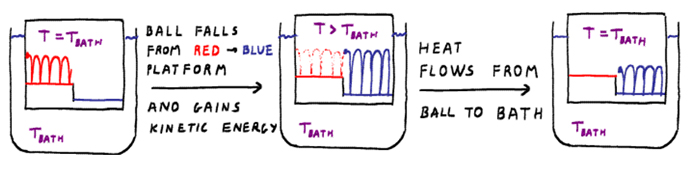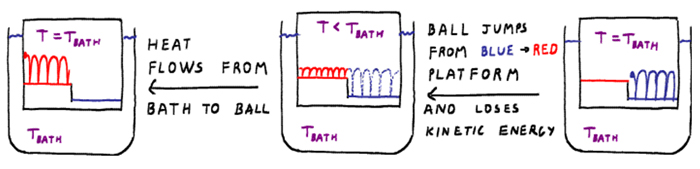# Entropy Activity

-Introduction

-The reaction coordinate

-The energy landscape

-Population distributions

-Motion at constant temperature, and exchange of energy with the heat bath

-Thermally activated processes

-Energy and temperature determine the populations

-Entropy and free energy

-Mathematical derivation of free energy

 < Previous | Next >

## Motion at constant temperature, and exchange of energy with the heat bath

In this activity, we explore the motion of molecules at a constant temperature.

Virtual Activity

- Motion at constant temperature, and exchange of energy with the heat bath (Java Required) [Opens in a separate window. Please read through all content in the lower window, using the scroll bar.]

In the above activity, you should have found that the average height of the particles on the two platforms is the same. This is an important aspect of molecular motion at constant temperature. The motion of particles is referred to as kinetic energy and temperature is a measure of the average kinetic energy of the atoms and molecules,where k is the "Boltzmann constant" with a value 1.3806503 x 10-23 J/K. (The units are Joules (energy) per Kelvin (degrees).) The heat bath ensures that the average kinetic energy of the particles is equal to 1/2 kT per degree of freedom.

Dynamics at constant temperature, i.e. on a shaking platform, is considerably different than everyday dynamics. Consider a ball bouncing on the above platforms. The height of the bounce sets the average kinetic energy of the ball. Suppose the ball falls off the upper platform and onto the lower platform, as shown below.When the ball falls to the lower platform, gravitational potential energy is converted into kinetic energy, so the degree of bouncing is higher on the lower platform than it was on the upper platform. This means the average kinetic energy of the ball has increased. Since temperature is directly proportional to the average kinetic energy, this means the temperature of the system has increased.

In contact with a heat bath (shaking platform), energy will flow into or out of the heat bath to keep the system at constant temperature. The heat bath is constantly giving and taking kinetic energy from the ball, in such a way that the average height of the bouncing ball remains constant. When the ball falls off the platform, transitioning from state 1 to state 2, the potential energy decreases. This energy initially appears as an increase in kinetic energy, corresponding to an increase in temperature. The temperature of the ball is now higher than that of the heat bath (T>Tbath), so heat flows into the heat bath until the temperature of the ball becomes equal to that of the heat bath (T=Tbath). As heat flows into the heat bath, the kinetic energy of the ball decreases until the average height of the bouncing is equal to that the ball initially had on the upper platform.As the ball moves from state 1 (upper platform) to state 2 (lower platform), heat flows into the heat bath. The opposite is true for a transition from state 2 to state 1. Following the figure below from right to left, we see that as the ball transitions from the lower to the upper platform, it loses kinetic energy and its temperature drops below that of the bath. Heat then flow into the system from the heat bath, increasing the average kinetic energy so that the ball is now bouncing with the same average height it initially had on the lower platform.Question: Supposed that the difference in energy between the upper and lower platform in the above diagrams is 5 kJ/mol. When the ball falls from the upper to lower platform, what is the flow of energy (both magnitude and direction) between the ball and the heat bath?

 < Previous | Next >